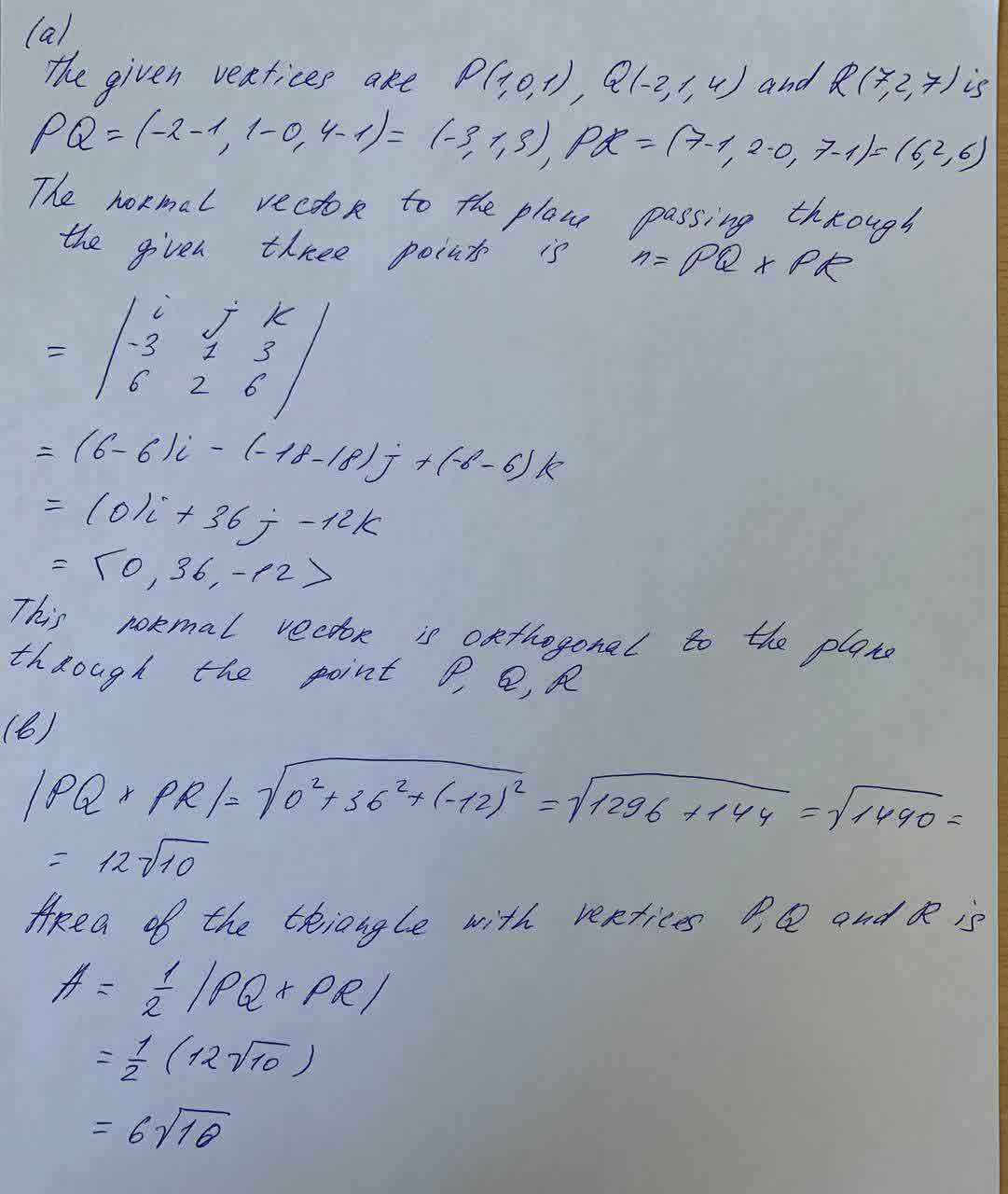# Find a nonzero vector orthogonal to the plane through the points P, Q, and R. and area of the triangle PQR Consider the points below P(1,0,1) , Q(-2,1,4) , R(7,2,7) a) Find a nonzero vector orthogonal to the plane through the points P,Q and R b) Find the area of the triangle PQRbeljuA 2021-05-14 Answered
Find a nonzero vector orthogonal to the plane through the points P, Q, and R. and area of the triangle PQR
Consider the points below
P(1,0,1) , Q(-2,1,4) , R(7,2,7)
a) Find a nonzero vector orthogonal to the plane through the points P,Q and R
b) Find the area of the triangle PQR
You can still ask an expert for help

• Questions are typically answered in as fast as 30 minutes

Solve your problem for the price of one coffee

• Math expert for every subject
• Pay only if we can solve itsaiyansruleA
Solution to your question with vector:###### Not exactly what you’re looking for?Jeffrey Jordon

a) The given vertices are $P\left(1,0,1\right),Q\left(-2,1,4\right)$ and $R\left(7,2,7\right)$ is

The normal vector to the plane passing through the given three points is $n=PQ×PR$

$=|\begin{array}{ccc}i& j& k\\ -3& 1& 3\\ 6& 2& 6\end{array}|$

$=\left(6-6\right)i-\left(-18-18\right)j+\left(-8-6\right)k$

$=\left(0\right)i+36j-12k$

$=<0,36,-12>$

This normal vector is orthogonal to the plane throught the point $P,Q,R$

b) $|PQ×PR|=\sqrt{{0}^{2}+{36}^{2}+\left(-12{\right)}^{2}}=\sqrt{1296+144}=\sqrt{1440}$

$=12\sqrt{10}$

Area of the triangle with verties $P,Q$ and $R$ is

$A=\frac{1}{2}|PQ×PR|$

$=\frac{1}{2}\left(12\sqrt{10}\right)$

$=6\sqrt{10}$